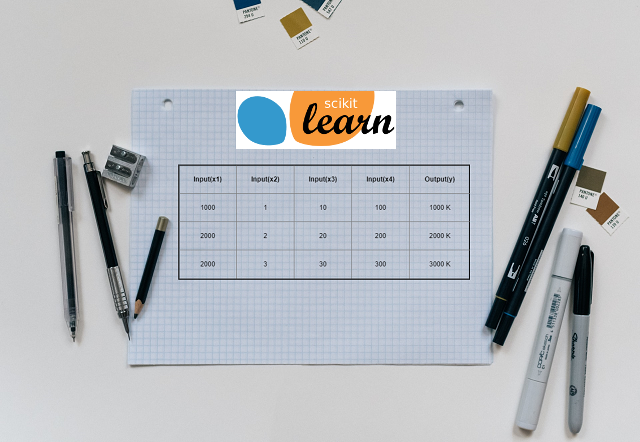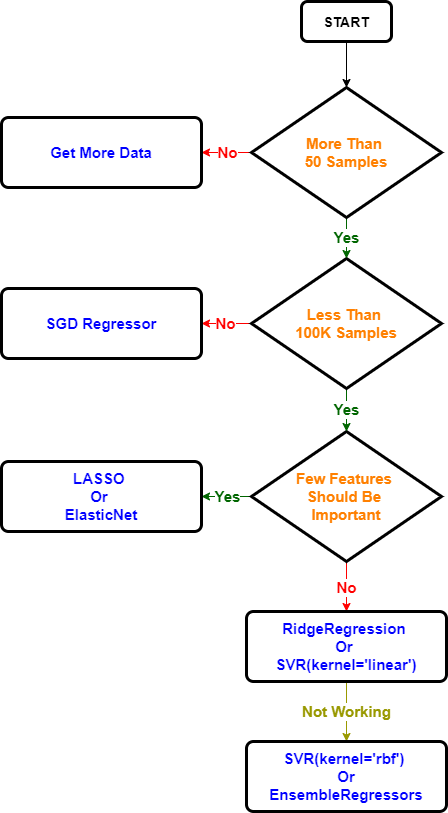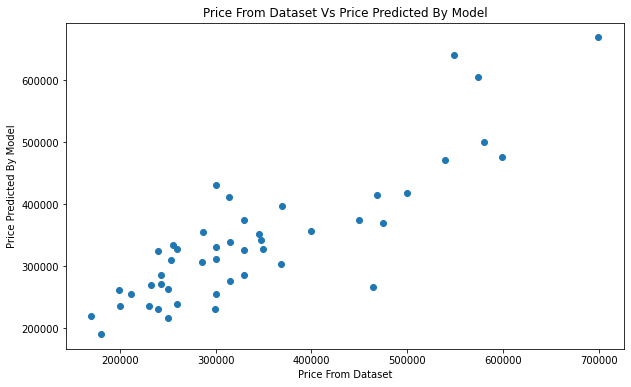# Multivariate Linear Regression Using Scikit LearnIn this tutorial we are going to use the Linear Models from Sklearn library. We are also going to use the same test data used in Multivariate Linear Regression From Scratch With Python tutorial

## Introduction

Scikit-learn is one of the most popular open source machine learning library for python. It provides range of machine learning models, here we are going to use linear model. Sklearn linear models are used when target value is some kind of linear combination of input value. Sklearn library has multiple types of linear models to choose form. The way we have implemented the ‘Batch Gradient Descent’ algorithm in Multivariate Linear Regression From Scratch With Python tutorial, every Sklearn linear model also use specific mathematical model to find the best fit line.

## Hypothesis Function Comparison

The hypothesis function used by Linear Models of Sklearn library is as below

y(w, x) = w_0 + (w_1 * x_1) + (w_2 * x_2) …….(w_n * x_n)

Where,

• y(w, x) = Target/output value
• x_1 to x_n = Dependent/Input value
• w_0 = intercept
• w_1 to w_n = as coef for every input feature(x_1 to x_n)

You must have noticed that above hypothesis function is not matching with the hypothesis function used in Multivariate Linear Regression From Scratch With Python tutorial. Actually both are same, just different notations are used

h(θ, x) = θ_0 + (θ_1 * x_1) + (θ_2 * x_2)……(θ_n * x_n)

Where,

• Both the hypothesis function use ‘x’ to represent input values or features
• y(w, x) = h(θ, x) = Target or output value
• w_0 = θ_0 = intercept or Y intercept
• w_1 to w_n = θ_1 to θ_n = coef or slope/gradient

## Python Code

Yes, we are jumping to coding right after hypothesis function, because we are going to use Sklearn library which has multiple algorithms to choose from.

## Import the required libraries

• numpy : Numpy is the core library for scientific computing in Python. It is used for working with arrays and matrices.
• pandas: Used for data manipulation and analysis
• matplotlib : It’s plotting library, and we are going to use it for data visualization
• linear_model: Sklearn linear regression model

In case you don’t have any experience using these libraries, don’t worry I will explain every bit of code for better understanding

``````import numpy as np
import pandas as pd
import matplotlib.pyplot as plt
from sklearn import linear_model
``````

## Load the data

• We are going to use ‘multivariate_housing_prices_in_portlans_oregon.csv’ CSV file
• File contains three columns ‘size(in square feet)’, ‘number of bedrooms’ and ‘price’
``````df = pd.read_csv('https://raw.githubusercontent.com/satishgunjal/datasets/master/multivariate_housing_prices_in_portlans_oregon.csv')
print('Dimension of dataset= ', df.shape)
df.head() # To get first n rows from the dataset default value of n is 5
``````
``````Dimension of dataset=  (47, 3)
``````
size(in square feet) number of bedrooms price
0 2104 3 399900
1 1600 3 329900
2 2400 3 369000
3 1416 2 232000
4 3000 4 539900
``````X = df.values[:, 0:2]  # get input values from first two columns
y = df.values[:, 2]  # get output values from last coulmn
m = len(y) # Number of training examples

print('Total no of training examples (m) = %s \n' %(m))

# Show only first 5 records
for i in range(5):
print('X =', X[i, ], ', y =', y[i])
``````
``````Total no of training examples (m) = 47

X = [2104    3] , y = 399900
X = [1600    3] , y = 329900
X = [2400    3] , y = 369000
X = [1416    2] , y = 232000
X = [3000    4] , y = 539900
``````

## Understand the data

• There are total 47 training examples (m= 47 or 47 no of rows)
• There are two features (two columns of feature and one of label/target/y)
• Total no of features (n) = 2

### Feature Normalization

• As you can notice size of the house and no of bedrooms are not in same range(house sizes are about 1000 times the number of bedrooms).
• Sklearn provides libraries to perform the feature normalization. We don’t have to write our own function for that.
• During model training we will enable the feature normalization
• To know more about feature normalization please refer ‘Feature Normalization’ section in Multivariate Linear Regression From Scratch With Python tutorial

## Which Sklearn Linear Regression Algorithm To Choose

• Sklearn library have multiple linear regression algorithms
• Note: The way we have implemented the cost function and gradient descent algorithm in previous tutorials every Sklearn algorithm also have some kind of mathematical model.
• Different algorithms are better suited for different types of data and type of problems
• Flow chart below will give you brief idea on how to choose right algorithm## Ordinary Least Squares Algorithm

• This is one of the most basic linear regression algorithm.
• Mathematical formula used by ordinary least square algorithm is as below,• The objective of Ordinary Least Square Algorithm is to minimize the residual sum of squares. Here the term residual means ‘deviation of predicted value(Xw) from actual value(y)’
• Problem with ordinary least square model is size of coefficients increase exponentially with increase in model complexity
``````model_ols =  linear_model.LinearRegression(normalize=True)
model_ols.fit(X,y)
# fit() method is used for training the model
# Note the first parameter(feature) is must be 2D array(feature matrix)
``````
``````LinearRegression(copy_X=True, fit_intercept=True, n_jobs=None, normalize=True)
``````

### Understanding Training Results

• Note: If training is successful then we get the result like above. Where all the default values used by LinearRgression() model are displayed.
• Note that ‘normalization = true’
• As per our hypothesis function, ‘model’ object contains the coef and intercept values
``````coef = model_ols.coef_
intercept = model_ols.intercept_
print('coef= ', coef)
print('intercept= ', intercept)
``````
``````coef=  [  139.21067402 -8738.01911233]
intercept=  89597.90954279757
``````

Note that for every feature we get the coefficient value. Since we have two features(size and no of bedrooms) we get two coefficients. Magnitude and direction(+/-) of all these values affect the prediction results.

### Visualization

• Check below table for comparison between price from dataset and predicted price by our model
• We will also plot the scatter plot of price from dataset vs predicted weight

Note: Here we are using the same dataset for training the model and to do predictions. Recommended way is to split the dataset and use 80% for training and 20% for testing the model. We will learn more about this in future tutorials.

``````predictedPrice = pd.DataFrame(model_ols.predict(X), columns=['Predicted Price']) # Create new dataframe of column'Predicted Price'
actualPrice = pd.DataFrame(y, columns=['Actual Price'])
actualPrice = actualPrice.reset_index(drop=True) # Drop the index so that we can concat it, to create new dataframe
df_actual_vs_predicted = pd.concat([actualPrice,predictedPrice],axis =1)
df_actual_vs_predicted.T
``````
0 1 2 3 4 5 6 7 8 9 10 11 12 13 14 15 16 17 18 19 20 21 22 23 24 25 26 27 28 29 30 31 32 33 34 35 36 37 38 39 40 41 42 43 44 45 46
Actual Price 399900.000000 329900.000000 369000.000000 232000.000000 539900.000000 299900.000000 314900.000000 198999.000000 212000.00000 242500.000000 239999.000000 347000.000000 329999.000000 699900.000000 259900.00000 449900.000000 299900.000000 199900.000000 499998.000000 599000.000000 252900.000000 255000.000000 242900.00000 259900.000000 573900.000000 249900.000000 464500.000000 469000.000000 475000.000000 299900.00000 349900.000000 169900.000000 314900.000000 579900.000000 285900.000000 249900.000000 229900.000000 345000.000000 549000.000000 287000.00000 368500.000000 329900.000000 314000.000000 299000.000000 179900.000000 299900.000000 239500.000000
Predicted Price 356283.110339 286120.930634 397489.469848 269244.185727 472277.855146 330979.021018 276933.026149 262037.484029 255494.58235 271364.599188 324714.540688 341805.200241 326492.026099 669293.212232 239902.98686 374830.383334 255879.961021 235448.245292 417846.481605 476593.386041 309369.113195 334951.623863 286677.77333 327777.175516 604913.374134 216515.593625 266353.014924 415030.014774 369647.335045 430482.39959 328130.300837 220070.564448 338635.608089 500087.736599 306756.363739 263429.590769 235865.877314 351442.990099 641418.824078 355619.31032 303768.432883 374937.340657 411999.633297 230436.661027 190729.365581 312464.001374 230854.293049
``````plt.scatter(y, model_ols.predict(X))
plt.xlabel('Price From Dataset')
plt.ylabel('Price Predicted By Model')
plt.rcParams["figure.figsize"] = (10,6) # Custom figure size in inches
plt.title("Price From Dataset Vs Price Predicted By Model")
``````## Testing the model

• Question: Estimate the price of a 1650 sq-ft, 3-bedroom house
• We can simply use ‘predict()’ of sklearn library to predict the price of the house
``````price = model_ols.predict([[2104,    3]])
print('Predicted price of a 1650 sq-ft, 3 br house:', price)
``````
``````Predicted price of a 1650 sq-ft, 3 br house: [356283.1103389]
``````

## Ridge Regression Algorithm

• Ridge regression addresses some problems of Ordinary Least Squares by imposing a penalty on the size of the coefficients
• Ridge model uses complexity parameter alpha to control the size of coefficients
• Note: alpha should be more than ‘0’, or else it will perform same as ordinary linear square model
• Mathematical formula used by Ridge Regression algorithm is as below,``````model_r = linear_model.Ridge(normalize= True, alpha= 35)
model_r.fit(X,y)
print('coef= ' , model_r.coef_)
print('intercept= ' , model_r.intercept_)
price = model_r.predict([[2104,    3]])
print('Predicted price of a 1650 sq-ft, 3 br house:', price)
``````
``````coef=  [   3.70764427 1958.37472904]
intercept=  326786.38211867993
Predicted price of a 1650 sq-ft, 3 br house: [340462.38984537]
``````

## LASSO Regression Algorithm

• Similar to Ridge regression LASSO also uses regularization parameter alpha but it estimates sparse coefficients i.e. more number of 0 coefficients
• That’s why its best suited when dataset contains few important features
• LASSO model uses regularization parameter alpha to control the size of coefficients
• Note: alpha should be more than ‘0’, or else it will perform same as ordinary linear square model
• Mathematical formula used by LASSO Regression algorithm is as below,``````model_l = linear_model.Lasso(normalize= True, alpha= 0.55)
model_l.fit(X,y)
print('coef= ' , model_l.coef_)
print('intercept= ' , model_l.intercept_)
price = model_l.predict([[2104,    3]])
print('Predicted price of a 1650 sq-ft, 3 br house:', price)
``````
``````coef=  [  139.19963776 -8726.55682971]
intercept=  89583.65169819258
Predicted price of a 1650 sq-ft, 3 br house: [356280.01905528]
``````

## Conclusion

As you can notice with Sklearn library we have very less work to do and everything is handled by library. We don’t have to add column of ones, no need to write our cost function or gradient descent algorithm. We can directly use library and tune the hyper parameters (like changing the value of alpha) till the time we get satisfactory results. If you are following my machine learning tutorials from the beginning then implementing our own gradient descent algorithm and then using prebuilt models like Ridge or LASSO gives us very good perspective of inner workings of these libraries and hopeful it will help you understand it better.

## Learning Path for DP-900 Microsoft Azure Data Fundamentals Certification

8 minute read

Learning path to gain necessary skills and to clear the Azure Data Fundamentals Certification. This certification is intended for candidates beginning to wor...

## Learning Path for AI-900 Microsoft Azure AI Fundamentals Certification

7 minute read

Learning path to gain necessary skills and to clear the Azure AI Fundamentals Certification. This certification is intended for candidates with both technica...

## ANN Model to Classify Images

12 minute read

In this guide we are going to create and train the neural network model to classify the clothing images. We will use TensorFlow deep learning framework along...

## Introduction to NLP

8 minute read

In short NLP is an AI technique used to do text analysis. Whenever we have lots of text data to analyze we can use NLP. Apart from text analysis, NLP also us...

## K Fold Cross Validation

14 minute read

There are multiple ways to split the data for model training and testing, in this article we are going to cover K Fold and Stratified K Fold cross validation...

## K-Means Clustering

13 minute read

K-Means clustering is most commonly used unsupervised learning algorithm to find groups in unlabeled data. Here K represents the number of groups or clusters...

## Time Series Analysis and Forecasting

10 minute read

Any data recorded with some fixed interval of time is called as time series data. This fixed interval can be hourly, daily, monthly or yearly. Objective of t...

## Support Vector Machines

9 minute read

Support vector machines is one of the most powerful ‘Black Box’ machine learning algorithm. It belongs to the family of supervised learning algorithm. Used t...

## Random Forest

12 minute read

Random forest is supervised learning algorithm and can be used to solve classification and regression problems. Unlike decision tree random forest fits multi...

## Decision Tree

13 minute read

Decision tree explained using classification and regression example. The objective of decision tree is to split the data in such a way that at the end we hav...

## Agile Scrum Framework

7 minute read

This tutorial covers basic Agile principles and use of Scrum framework in software development projects.

## Underfitting & Overfitting

2 minute read

Main objective of any machine learning model is to generalize the learning based on training data, so that it will be able to do predictions accurately on un...

## Multiclass Logistic Regression Using Sklearn

6 minute read

In this study we are going to use the Linear Model from Sklearn library to perform Multi class Logistic Regression. We are going to use handwritten digit’s d...

## Binary Logistic Regression Using Sklearn

6 minute read

In this tutorial we are going to use the Logistic Model from Sklearn library. We are also going to use the same test data used in Logistic Regression From Sc...

## Logistic Regression From Scratch With Python

14 minute read

This tutorial covers basic concepts of logistic regression. I will explain the process of creating a model right from hypothesis function to algorithm. We wi...

## Train Test Split

3 minute read

In this tutorial we are going to study about train, test data split. We will use sklearn library to do the data split.

## One Hot Encoding

11 minute read

In this tutorial we are going to study about One Hot Encoding. We will also use pandas and sklearn libraries to convert categorical data into numeric data.

## Multivariate Linear Regression Using Scikit Learn

8 minute read

In this tutorial we are going to use the Linear Models from Sklearn library. Scikit-learn is one of the most popular open source machine learning library for...

## Univariate Linear Regression Using Scikit Learn

7 minute read

In this tutorial we are going to use the Linear Models from Sklearn library. Scikit-learn is one of the most popular open source machine learning library for...

## Multivariate Linear Regression From Scratch With Python

10 minute read

In this tutorial we are going to cover linear regression with multiple input variables. We are going to use same model that we have created in Univariate Lin...

## Univariate Linear Regression From Scratch With Python

13 minute read

This tutorial covers basic concepts of linear regression. I will explain the process of creating a model right from hypothesis function to gradient descent a...

## Machine Learning Introduction And Learning Plan

4 minute read

In this tutorial we will see the brief introduction of Machine Learning and preferred learning plan for beginners

Back to top ↑